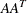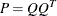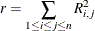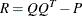### Example 5.1 Matrix Square Root

This example demonstrates the use of PROC OPTMODEL array parameters and variables. The following statements create a randomized positive definite symmetric matrix and define an optimization model to find the matrix square root of the generated matrix:

proc optmodel;
number n = 5;  /* size of matrix */
/* random original array */
number A{1..n, 1..n} = 10 - 20*ranuni(-1);
/* compute upper triangle of the
* symmetric matrix A*transpose(A) */
/* should be positive def unless A is singular */
number P{i in 1..n, j in i..n};
for {i in 1..n, j in i..n}
P[i,j] = sum{k in 1..n} A[i,k]*A[j,k];
/* coefficients of square root array
* (upper triangle of symmetric matrix) */
var q{i in 1..n, i..n};
/* The default initial value q[i,j]=0 is
* a local minimum of the objective,
* so you must move it away from that point. */
q[1,1] = 1;
/* minimize difference of square of q from P */
min r = sum{i in 1..n, j in i..n}
(   sum{k in 1..i} q[k,i]*q[k,j]
+ sum{k in i+1..j} q[i,k]*q[k,j]
+ sum{k in j+1..n} q[i,k]*q[j,k]
- P[i,j] )**2;
solve;
print q;


These statements define a random array A of size. The product P is defined as the matrix product. The product is symmetric, so the declaration of the parameter P gives it upper triangular indexing. The matrix represented by P should be positive definite unless A is singular. But singularity is unlikely because of the random generation of A. If P is positive definite, then it has a well-defined square root, Q, such that.

The objective r simply minimizes the sum of squares of the coefficients aswhere. (This technique for computing matrix square roots is intended only for the demonstration of PROC OPTMODEL capabilities. Better methods exist.)

Output 5.1.1 shows part of the output from running these statements. The values that are actually displayed depend on the random numbers generated.

Output 5.1.1: Matrix Square Root Results

q
1 2 3 4 5
1 12.67867 -8.14753 -6.43848 -0.87666 1.46609
2   -2.45955 -8.23167 4.22369 -8.64930
3     9.20976 2.70390 2.31570
4       -2.41761 -2.44853
5         7.22670